# Texas Go Math Grade 1 Lesson 4.3 Answer Key Model Putting Together

Refer to our Texas Go Math Grade 1 Answer Key Pdf to score good marks in the exams. Test yourself by practicing the problems from Texas Go Math Grade 1 Lesson 4.3 Answer Key Model Putting Together.

## Texas Go Math Grade 1 Lesson 4.3 Answer Key Model Putting Together

Essential Question
How can you model putting together, or joining?
Explanation:
For adding the numbers putting together is used.

Explore
Select, real objects, or an ¡Tool to model the problem. Draw a picture of your model. Write the numbers and the addition sentence.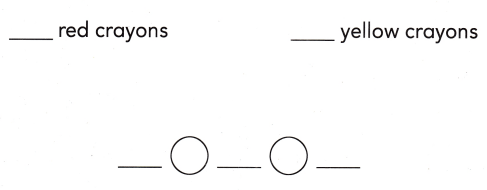There are ___ crayons.Explanation:
There are 5 crayons.

For The Teacher

• Read the following problem. There are 2 red crayons and 3 yellow crayons. How many crayons are there?

Math Talk
Mathematical Processes
Describe how the tool you selected helped you solve.
Explanation:
It shows how many are here individually

Model and Draw

Add to find how many books there are.

There are 2 small books and 1 big book. How many books are there?
____ books ____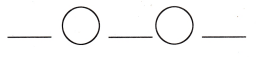Explanation:
2 + 1 = 3 Books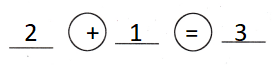Share and Show

Useto solve. Draw to show your work.
Write the number sentence and how many.

Question 1.
There are 4 red pencils and 2 green pencils. How many pencils are there?
___ pencils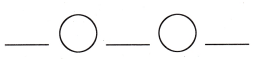Explanation:
4 + 2 = 6Question 2.
There are 5 blue scissors and 3 yellow scissors. How many scissors are there?
____ scissorsExplanation:
5 + 3 = 8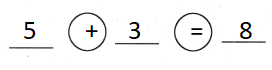Problem Solving

Useto solve. Draw to show your work.
Write the number sentence and how many.

Question 3.
There are 3 small cats and 4 big cats. How many cats are there?
____ cats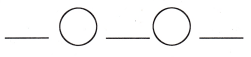Explanation: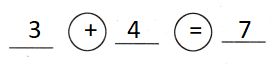Write or draw to solve.

Question 4.
Multi-Step 4 girls and 4 boys run. Then 2 more children run. The same number of girls and boys run. How many of each run?Explanation:
4 + 1 = 5 boys
4 + 1 = 5 girls
Each run 5

Question 5.
H.O.T. There are 2 red cups and some green cups. There are 10 cups. How many cups are green?
____ green cupsExplanation:
8 + 2 = 10
8 cups are green

Question 6.
A penguin uses 5 stones to make a nest. Another penguin uses 4 stones to make a nest. How many stones do the penguins use?
(A) 1
(B) 9
(C) 6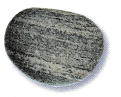Explanation:
4 + 5 = 9
9 stones that the penguins use

Question 7.
Use Tools There are 7 children at the park. 2 adults are also there. How many children and adults are at the park?
(A) 9
(B) 7
(C) 2
Explanation:
7 + 2 = 9
9 children and adults are at the park

Question 8.
Multi-Step There are 6 birds sitting on their nests. There are 2 turtles and 3 frogs swimming in the pond. How many animals are there?
(A) 11
(B) 8
(C) 5
Explanation:
6 + 2 + 3 = 11
11 animals are there

Question 9.
Texas Test Prep Use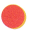to solve. There are 7 yellow apples and 3 green apples. How many apples are there?
(A) 4
(B) 9
(C) 10
Explanation:
7 + 3 = 10
10 apples are there

Take Home Activity

• Have your child collect a group of up to 10 small objects, and use them to make up addition stories.

### Texas Go Math Grade 1 Lesson 4.3 Homework and Practice Answer Key

Use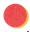to solve. Draw to show your work. Write the number sentence and how many.

Question 1.
Mike sees 2 blue birds and 7 red birds. How many birds does he see?
____ birds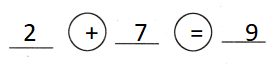Explanation:
2 + 7 = 9
9 birds that  he see

Problem Solving

Select, real objects, or an iTool to solve.

Question 2.
Jack does 5 jumping jacks and some sit-ups. He does 10 exercises. How many sit-ups does he do?
___ sit-ups
Explanation:
5 + 5 = 10
5 sit-ups that he do

Question 3.
Multi-Step Lucy makes 1 bracelet and 3 rings. Then she makes 4 more items. Now she has the same number of bracelets and rings. How many of each does she make?
___ bracelets
____ rings
4 bracelets
4 rings
Explanation:
4 + 4 = 8
1 + 3 = 4
3 + 1 = 4

Question 4.
A painter uses 3 colors in one painting. He uses 6 different colors in another painting. How many colors does he use?
(A) 6
(B) 9
(C) 3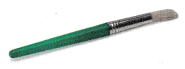Explanation:
3 + 6 = 9
9 colors that he use

Question 5.
There are 2 girls in art class. There are 8 boys in art class. How many children are in art class?
(A) 9
(B) 6
(C) 10
Explanation:
2 + 8 = 10
10 children are in art class

Question 6.
There are 4 children in the play. There are 6 adults in the play. How many people are in the play?
(A) 2
(B) 10
(C) 6
Explanation:
4 + 6 = 10
10 people are in the play

Question 7.
Multi-Step A writer sells 4 stories and 2 poems at the street fair. A painter sells 2 paintings. How many items are sold at the street fair? ,
(A) 8
(B) 2
(C) 6